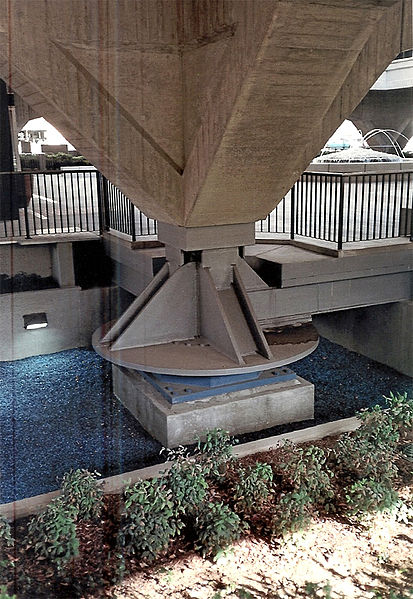# 结构力学中阻尼的来源及数学理论

2019年 3月 14日

### 如何定量计算阻尼？m \ddot u + c\dot u + k u = 0

\ddot u + 2 \zeta \omega_0 \dot u + \omega_o^2 u = 0

e^{-\zeta \omega_0 t} = e^{\frac{-2 \pi \zeta t }{T_0}}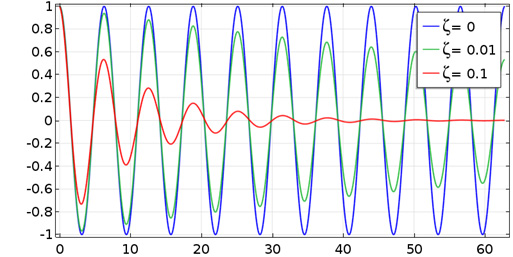\delta = \mathrm {ln} \left ( \dfrac{u(t_i)}{u(t_{i+1})} \right ) = \mathrm {ln} \left ( \dfrac{u(t_i)}{u(t_i+T)}\right )

\delta = \dfrac{2 \pi \zeta}{\sqrt{1-\zeta^2}} \approx 2 \pi \zetaQ = \dfrac{1}{2 \zeta \sqrt{1-\zeta^2}} \approx \dfrac{1}{2 \zeta}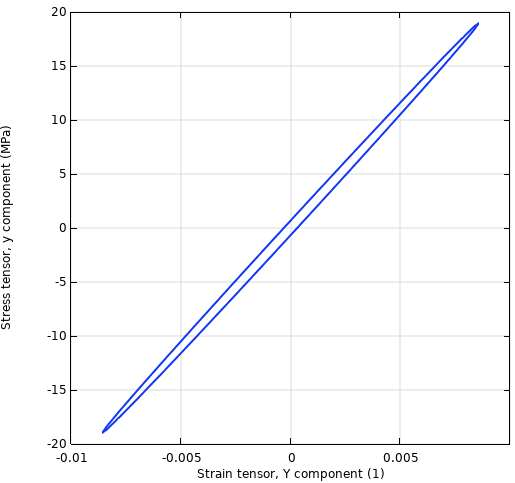\tilde \sigma = \tilde E \tilde \varepsilon = (E^\prime+iE^{\prime \prime}) \tilde \varepsilon

\tilde E = E(1+i \eta)

E = |\tilde E| = \sqrt{(E^\prime)^2+(E{\prime \prime})^2}

\tilde E = \dfrac{E(1+i \eta)}{\sqrt{1+\eta^2}}

\mathrm {tan} \, \delta = \dfrac{E^{\prime \prime}}{E^\prime} = \eta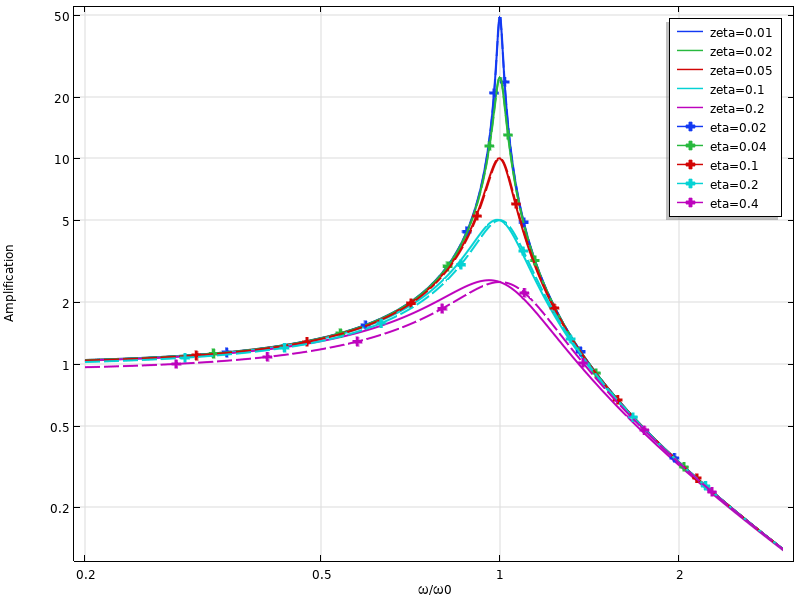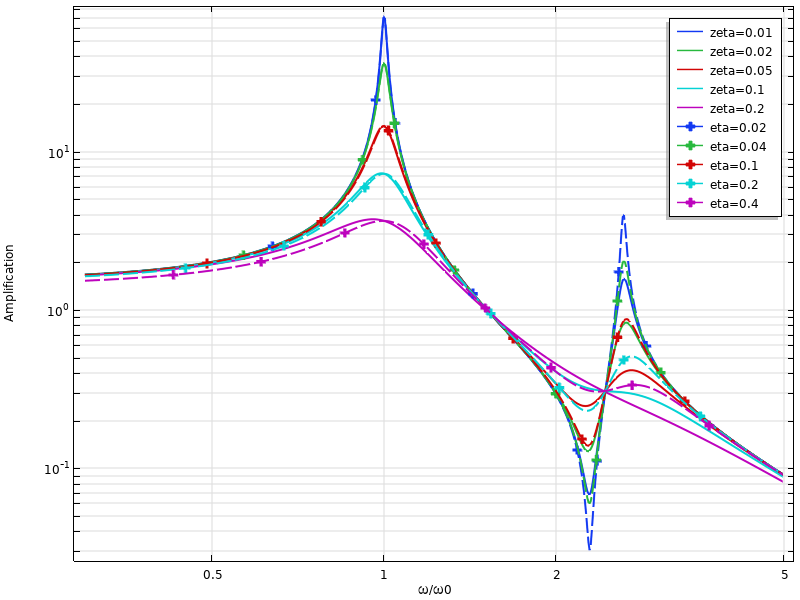D = \pi E^{\prime \prime} \varepsilon_a^2

W_s = \dfrac{1}{2}E^{\prime} \varepsilon_a^2

\eta = \dfrac{E^{\prime \prime}}{E^\prime} = \dfrac{D}{2 \pi W_s}

### 阻尼的来源

0.0001–0.02

0.001–0.05

0.0001–0.01

#### 声音发射Tonpilz 换能器中的声辐射图。

#### 缓冲器#### 减震器